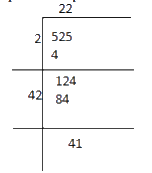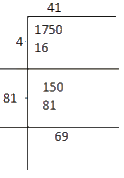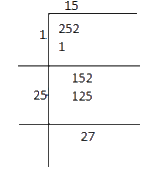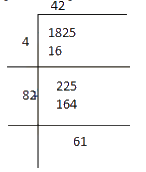# Ex.6.4 Q5 Squares and Square Roots - NCERT Maths Class 8

## Question

Find the least number which must be added to each of the following numbers so as to get a perfect square. Also find the square root of the perfect square so obtained.

(i) $$525$$

(ii) $$1750$$

(iii) $$252$$

(iv) $$1825$$

(v) $$6412$$

Video Solution
Squares And Square Roots
Ex 6.4 | Question 5

## Text Solution

What is known?

Numbers that are not perfect square

What is unknown?

What must be added to the numbers so as to get perfect square

Steps:

(i)

Square root of $$525$$ is calculated by long division method as follows.It is evident that $${22^2} < 525$$

Next, the perfect square of $$23$$ is

$${23^2} = 529$$

Hence, the number to be added to $$529$$

\begin{align}&= {23^2} - 525\\&= 529 - 525\\&= 4\end{align}

The required perfect square is $$525 + 4 = 529$$

$$\sqrt {529}= 23$$

(ii)

Square root of $$1750$$ can be calculated by the long division method as follows.The remainder is $$69$$.

This shows that $${41^2} < 1750$$

Next perfect square is $${42^2} = 1764$$

Hence number to be added to $$1750$$

\begin{align}&= {42^2} - 1750\\&= 1764 - 1750\\&= 14\end{align}

The required perfect square is $$1750 + 14 = 1764$$

$$\sqrt {1764}= 42$$

(iii)

Square root of $$252$$ is calculated as follows.The remainder is $$27.$$

This shows that $${15^2} < 252$$

Next perfect square is $${16^2} = 256$$

Hence number to be added to $$252$$

\begin{align}&= {16^2} - 252\\&= 256 - 252\\&= 4\end{align}

The required perfect square is $$252 + 4 = 256$$

$${\rm{}}\;\sqrt {256}= 16$$

(iv)Square root of $$1825$$ is calculated as follows.

The remainder is $$61.$$

This shows that $${42^2} < 1825$$

Next perfect square is $${43^2} = 1849$$

Hence number to be added to $$1825$$

\begin{align}&= {43^2} - 1825\\&= 1849 - 1825\\&= 24\end{align}

The required perfect square is $$1825 + 24 = 1849$$

$${\rm{And}}\;\sqrt {1849}= 43$$

(v)

Square root of $$6412$$ is calculated as follows.$${80^2} < 6412$$The remainder is $$12.$$This shows that

Next perfect square is $${81^2} = 6561$$

Hence number to be added to $$6412$$ is

\begin{align}&= {16^2} - 252\\&= 256 - 252\\&= 4\end{align}

The required perfect square is $$6412 + 149 = 6561$$

$${\rm{And}}\;\sqrt {6561}= 81$$

Learn from the best math teachers and top your exams

• Live one on one classroom and doubt clearing
• Practice worksheets in and after class for conceptual clarity
• Personalized curriculum to keep up with school